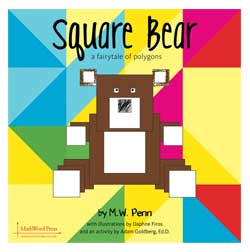# Lesson Plan for Square Bear

A Lesson Plan for Square BearCommon Core State Standard addressed in this lesson:
Identify and describe shapes (squares, circles, triangles, rectangles, hexagons, cubes, cones, cylinders, and spheres).

K.G.2. Correctly name shapes regardless of their orientations or overall size.

K.G.3. Identify shapes as two-dimensional (lying in a plane, “flat”) or three-dimensional (“solid”).

Analyze, compare, create, and compose shapes.

K.G.4. Analyze and compare two- and three-dimensional shapes, in different sizes and orientations, using informal language to describe their similarities, differences, parts (e.g., number of sides and vertices/“corners”) and other attributes (e.g., having sides of equal length).

K.G.5. Model shapes in the world by building shapes from components (e.g., sticks and clay balls) and drawing shapes.

K.G.6. Compose simple shapes to form larger shapes. For example, “Can you join these two triangles with full sides touching to make a rectangle?”

Reason with shapes and their attributes.

1.G.1 Distinguish between defining attributes (e.g., triangles are closed and three-sided) versus non-defining attributes (e.g., color, orientation, overall size); build and draw shapes to possess defining attributes.

1.G.2 Compose two-dimensional shapes (rectangles, squares, trapezoids, triangles, half-circles, and quarter-circles) or three-dimensional shapes (cubes, right rectangular prisms, right circular cones, and right circular cylinders) to create a composite shape, and compose new shapes from the composite shape.1

1.G.3 Partition circles and rectangles into two and four equal shares, describe the shares using the words halves, fourths, and quarters, and use the phrases half of, fourth of, and quarter of. Describe the whole as two of, or four of the shares. Understand for these examples that decomposing into more equal shares creates smaller shares.

Lesson Plan:
You will need: a copy of the book. For each student or group of students have one sheet of yellow paper; glue; a few sheets of many different triangles drawn on different colored construction paper.

To create these triangles, begin with another polygon and cut it into triangles: a square; a rectangle; a regular pentagon; a regular hexagon. (There is a sheet of polygons at the end of this lesson plan that can be used to make triangles.) First, cut out squares and then cut each in half to form two isosceles triangles. Next, cut out a rectangle and cut it in half to form two right triangles. Cut out a hexagon and cut it into four triangles. Continue with any regular polygons the students are studying: pentagons; octagons; etc. Now copy the triangles onto construction paper.

When you distribute the sheets of triangles to the students, mix the colors of the sheets, so that if students compose a square, it could be composed of two different colored triangles.

New words: Plane (flat, like the surface of the yellow paper); shapes in a plane (two-dimensional shapes); polygon.

Anticipatory Set and Objective: Read the story of Square Bear. The activity at the back of the book cuts polygons into triangles. Talk about building polygons from triangles. Using the triangles you’ve made, construct examples for the children.

Tell the students they are going to create two more characters to live in Polygon Palace with Square Bear. Each character will be constructed using only triangles, but the character must be made of shapes that are not triangles. For example, two triangles may be used to make a rectangle, three triangles can be used to make a pentagon, or four triangles may be used to make a hexagon.

Guided Practice: Give students sheets of triangles. They should cut out the triangles you gave them and use them to create characters. They will paste these characters on the ‘flat’ yellow ‘rectangle’ paper.

Emphasize the words flat and rectangle often, as children learn to distinguish two dimensional shapes in a plane as opposed to ‘solid’ figures.

When their characters are complete, have them count the number of shapes they used to make the character. Next have them count the number of triangles they used. They should write these numbers at the bottom of the yellow paper. Then they should create and write a name for their character.

Check for Understanding: Hang the characters around the room and compare the shapes the children used to make them.

Extension: As an extension, children can write a story that includes different characters of their choosing.